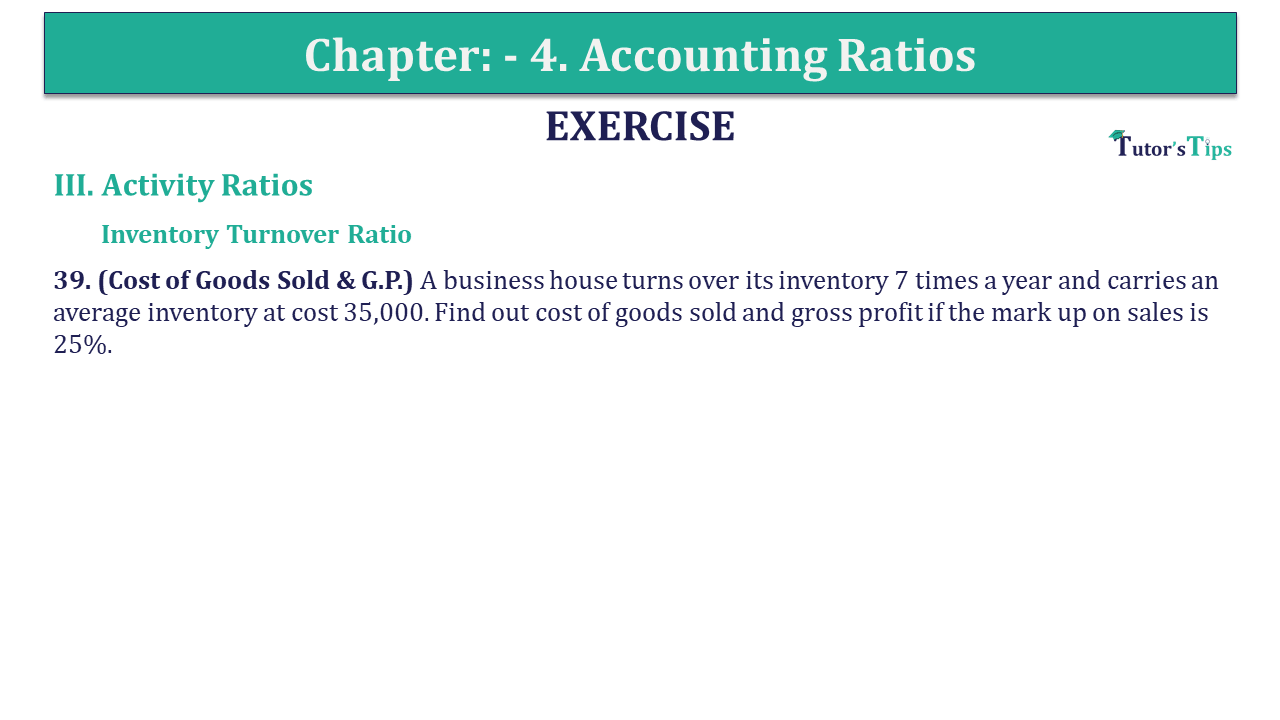# Question 39 Chapter 4 of +2-B – USHA Publication 12 ClassQ-39- CH-4 Book 2 - Usha Pub. +2 Book 2020 - Solution

Question 39 Chapter 4 of +2-B

III. Activity Ratios

Inventory Turnover Ratio

39. (Cost of Goods Sold & G.P.) A business house turns over its inventory 7 times a year and carries an average inventory at a cost of 35,000. Find out the cost of goods sold and gross profit if the mark upon sales is 25%.

## The solution of Question 39 Chapter 4 of +2-B: –

 Inventory Turnover Ratio = Cost of goods sold Average Inventory
 7 = Cost of goods sold ₹ 35,000
 Cost of goods sold = ₹ 35,000 x 7 = ₹ 2,45,000 Gross Profit on Sales = 25% Sales = ₹ 100 Cost of goods sold = 100 – 25 = 75
 Gross profit = ₹ 2,45,000 X 25 75 = ₹ 81,667

Also, Check out the solved question of previous Chapters: –